# C++数组的概念,一维数组，二维数组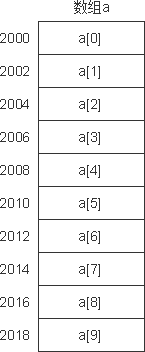C++用方括号来表示下标，如用s，s，s 分别代表s1，s2，s3。

## 定义一维数组

类型标识符  数组名[常量表达式];

int a;

1) 数组名定名规则和变量名相同，遵循标识符定名规则。

2) 用方括号括起来的常量表达式表示下标值，如下面的写法是合法的：
int a;
int a[2*5];
int a[n*2]; //假设前面已定义了n为常变量

3) 常量表达式的值表示元素的个数，即数组长度。例如，在“int a;”中，10表示a数组有10个元素，下标从0开始，这10个元素是： a，a，a，a，a，a，a，a， a，a。注意最后一个元素是a而不是a。

4) 常量表达式中可以包括常量、常变量和符号常量，但不能包含变量。也就是说，C++不允许对数组的大小作动态定义，即数组的大小不依赖于程序运行过程中变量的值。例如，下面这样定义数组是不行的：
int n;
cin>>n;  //输入a数组的长度
int a[n]; //企图根据n的值决定数组的长度

const int n=5;

## 引用一维数组的元素

数组名[下标]

a= a+ a- a[2*3]

【例5.1】数组元素的引用。
```#include <iostream>
using namespace std;
int main( )
{
int i, a;
for (i=0;i<=9;i++)
a[i]=i;
for (i=9;i>=0;i--)
cout<<a[i]<<" ";
cout<<endl;
return 0;
}```

9 8 7 6 5 4 3 2 1 0

## 一维数组的初始化

1) 在定义数组时分别对数组元素赋予初值。例如：
int a={0,1,2,3,4,5,6,7,8,9};

2) 可以只给一部分元素赋值。例如：
int a={0,1,2,3,4};

3) 如果想使一个数组中全部元素值为1，可以写成：
int a={1,1,1,1,1,1,1,1,1,1};

int a={1*10};

4) 在对全部数组元素赋初值时，可以不指定数组长度。例如：
int a={1,2,3,4,5};

int a[]={1,2,3,4,5};

## 一维数组程序举例

【例5.2】用数组来处理求Fibonacci数列问题。

```#include <iostream>
#include <iomanip>
using namespace std;
int main( )
{
int i;
int f={1,1}; //f=1,f=1
for(i=2;i<20;i++)
f[i]=f[i-2]+f[i-1]; //在i的值为2时,f=f+f,依此类推
for(i=0;i<20;i++) //此循环的作用是输出20个数
{
if(i%5==0) cout<<endl; //控制换行,每行输出5个数据
cout<<setw(8)<<f[i]; //每个数据输出时占8列宽度
}
cout<<endl; //最后执行一次换行
return 0;
}```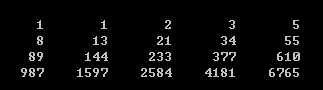【例5.3】编写程序，用起泡法对10个数排序（按由小到大顺序）。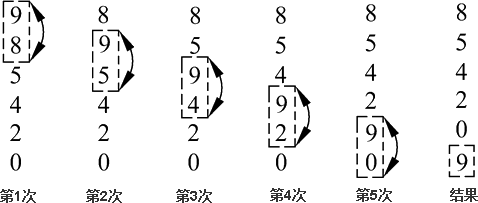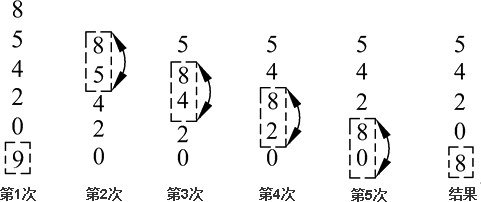```#include <iostream>
using namespace std;
int main( )
{
int a;
int i, j, t;
cout<<"input 10 numbers ："<<endl;
for (i=1;i<11;i++)  //输入a~a
cin>>a[i];
cout<<endl;
for (j=1;j<=9;j++) //共进行9趟比较
for(i=1;i<=10-j;i++)//在每趟中要进行(10-j)次两两比较
if (a[i]>a[i+1]) //如果前面的数大于后面的数
{
t=a[i];a[i]=a[i+1];a[i+1]=t;
}//交换两个数的位置, 使小数上浮
cout<<"the sorted numbers ："<<endl;
for(i=1;i<11;i++) //输出10个数
cout<<a[i]<<" ";
cout<<endl;
return 0;
}```

input 10 numbers：
3 5 9 11 33 6 -9 -76 100 123↙
the sorted numbers：
-76 -9 3 5 6 9 11 33 100 123

 学生序号 课程1 课程2 课程3 课程4 课程5 学生1 85 78 99 96 88 学生2 76 89 75 97 75 学生3 64 92 90 73 56

## 定义二维数组

类型标识符 数组名[常量表达式][常量表达式];

float a, b;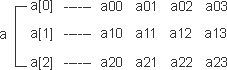float a, a, a;

C++中，二维数组中元素排列的顺序是：按行存放，即在内存中先顺序存放第一行的元素，再存放第二行的元素。图5.5表示对a数组存放的顺序。C++允许使用多维数组。有了二维数组的基础，再掌握多维数组是不困难的。例如，定义三维数组的方法是：
float a;

a→a→a→a→a→a→a→a→a→a→a→a→a→a→a→a→a→a→a→a→a→a→a→a

## 二维数组的引用

数组名 [下标][下标]

a

b=a/2;

int a;  //定义3行4列的数组
┆
a=15; //引用a元素

## 二维数组的初始化

1) 分行给二维数组赋初值。如
int a={{1,2,3,4},{5,6,7,8},{9,10,11,12}};

2) 可以将所有数据写在一个花括号内，按数组排列的顺序对各元素赋初值。如
int a={1,2,3,4,5,6,7,8,9,10,11,12};

3) 可以对部分元素赋初值。如：
int a={{1},{5},{9}};

1  0  0  0
5  0  0  0
9  0  0  0

int a={{1},{0,6},{0,0,11}};

1  0  0  0
0  6  0  0
0  0 11  0

int a={{1},{5,6}};

1  0  0  0
5  6  0  0
0  0  0  0

int a={{1},{},{9}};

4) 如果对全部元素都赋初值（即提供全部初始数据），则定义数组时对第一维的长度可以不指定，但第二维的长度不能省。如：
int a={1,2,3,4,5,6,7,8,9,10,11,12};

int a[]={1,2,3,4,5,6,7,8,9,10,11,12};

int a[]={{0,0,3},{},{0,10}};

0  0  3  0
0  0  0  0
0 10 0  0

C++在定义数组和表示数组元素时采用a[][]这种两个方括号的方式，对数组初始化时十分有用，它使概念清楚，使用方便，不易出错。

## 二维数组程序举例

【例5.4】将一个二维数组行和列元素互换，存到另一个二维数组中。例如：1. #include <iostream>
2. using namespace std;
3. int main( )
4. {
5. int a={{1,2,3},{4,5,6}};
6. int b,i,j;
7. cout<<"array a："<<endl;
8. for (i=0;i<=1;i++)
9. {
10. for (j=0;j<=2;j++)
11. {
12. cout<<a[i][j]<<" ";
13. b[j][i]=a[i][j];
14. }
15. cout<<endl;
16. }
17. cout<<"array b："<<endl;
18. for (i=0;i<=2;i++)
19. {
20. for(j=0;j<=1;j++)
21. cout<<b[i][j]<<" ";
22. cout<<endl;
23. }
24. return 0;
25. }

array a：
1  2  3
4  5  6
array b：
1  4
2  5
3  6

【例5.5】有一个3×4的矩阵，要求编程序求出其中值最大的那个元素的值，以及其所在的行号和列号。

1. #include <iostream>
2. using namespace std;
3. int main( )
4. {
5. int i,j,row=0,colum=0,max;
6. int a={{5,12,23,56},{19,28,37,46},{-12,-34,6,8}};
7. max=a;//使max开始时取a的值
8. for (i=0;i<=2;i++)//从第0行~第2行
9. for (j=0;j<=3;j++) //从第0列~第3列
10. if (a[i][j]>max) //如果某元素大于max
11. {
12. max=a[i][j]; //max将取该元素的值
13. row=i; //记下该元素的行号i
14. colum=j;//记下该元素的列号j
15. }
16. cout<<"max="<<max<<",row="<<row<<",colum="<<colum<<endl;
17. return 0;
18. }

max=56，row=0，colum=3

(2)
100%

(0)
0%